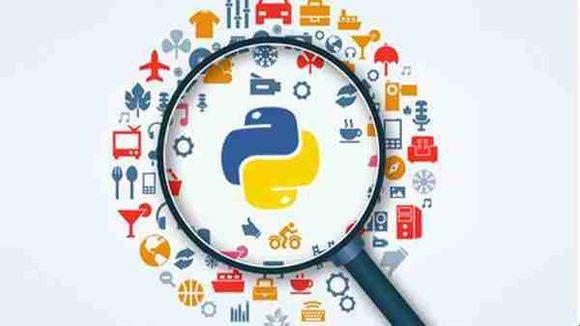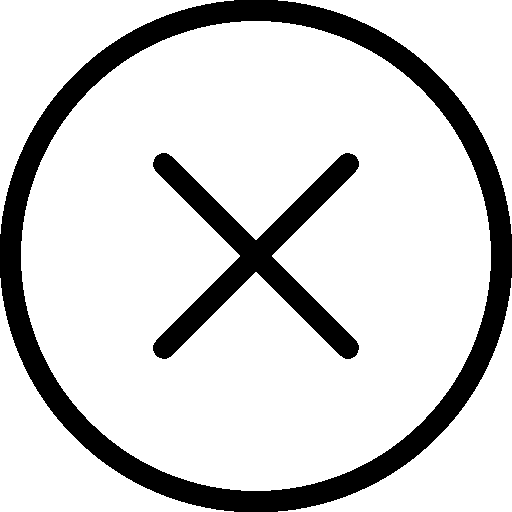• 写文章
• 提问

# Python3时间戳如何应用于数学计算？```# 文件名：count_days.py
import time
import datetime
def time_controller(d=0, hour=0, minute=0, second=0, arg=1, day=datetime.datetime.now()):
# 获得某天的x天、y小时、t分钟、z秒后的日期, day为空默认为当前时间开始
# 所有参数默认都为0，只需要调你需要的时间即可
# arg = 1: 获得hour小时,minute分钟,second秒后的具体时间
# arg = -1: 获得hour小时,minute分钟,second秒前的具体时间
now = datetime.datetime.now()
if type(day) == str:
now = datetime.datetime.strptime(day, '%Y-%m-%d %H:%M:%S')
if arg == 1:
value = now + datetime.timedelta(days=d, hours=hour, minutes=minute, seconds=second)
elif arg == -1:
value = now - datetime.timedelta(days=d, hours=hour, minutes=minute, seconds=second)
return value
print(time_controller(d=10, hour=20, minute=10, second=0, arg=-1, day='2019-08-01 10:00:00'))
print(time_controller(d=10, hour=20, minute=10, second=0, arg=-1))```

`python count_days.py`